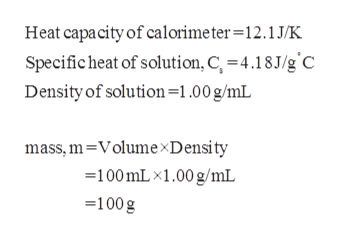# A 50.0 mL sample of a 1.00 M solution of CuSO4 is mixed with 50.0 mL of 2.00 M KOH in a calorimeter. The temperature of both solutions was 23.5 degrees (C)  before mixing and 31.0 degrees(C)  after mixing. The heat capacity of the calorimeter is 12.1 J/K.  From these data, calculate ΔH for the process:CuSO4(1M)+ 2KOH(2M) →Cu(OH)2(s) + K2SO4(0.5M)Assume that the specific heat and density of the solution after mixing are the same as those of pure water and that the volumes are additive.

Question
357 views

A 50.0 mL sample of a 1.00 M solution of CuSO4 is mixed with 50.0 mL of 2.00 M KOH in a calorimeter. The temperature of both solutions was 23.5 degrees (C)  before mixing and 31.0 degrees(C)  after mixing. The heat capacity of the calorimeter is 12.1 J/K.  From these data, calculate ΔH for the process:

CuSO4(1M)+ 2KOH(2M) →Cu(OH)2(s) + K2SO4(0.5M)

Assume that the specific heat and density of the solution after mixing are the same as those of pure water and that the volumes are additive.

check_circle

Step 1

Given,

Volume of CuSO4 = 50.0mL.

Volume of KOH = 50.0mL.

Initial temperature = 23.5 degree Celsius

Final temperature = 31.0 degree Celsius.

Step 2

Given that values of specific heat and density...help_outlineImage TranscriptioncloseHeat capacity of calorimeter=12.1J/K Specific heat of solution, C, 4.18J/g C Density of solution=1.00 g/mL mass,m-VolumexDensity 100mLx1.00 g/mL 100g fullscreen

### Want to see the full answer?

See Solution

#### Want to see this answer and more?

Solutions are written by subject experts who are available 24/7. Questions are typically answered within 1 hour.*

See Solution
*Response times may vary by subject and question.
Tagged in

### Physical Chemistry